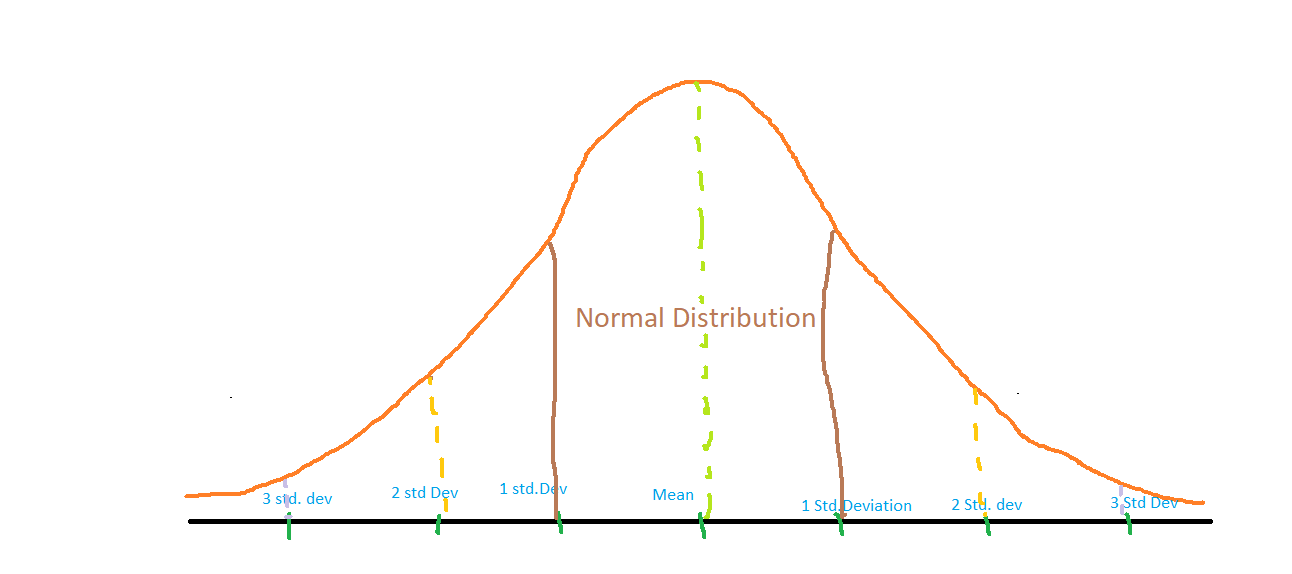# Z score for Outlier Detection – Python

Z score is an important concept in statistics. Z score is also called standard score. This score helps to understand if a data value is greater or smaller than mean and how far away it is from the mean. More specifically, Z score tells how many standard deviations away a data point is from the mean.

Z score = (x -mean) / std. deviation

A normal distribution is shown below and it is estimated that
68% of the data points lie between +/- 1 standard deviation.
95% of the data points lie between +/- 2 standard deviation
99.7% of the data points lie between +/- 3 standard deviationZ score and Outliers:
If the z score of a data point is more than 3, it indicates that the data point is quite different from the other data points. Such a data point can be an outlier.
For example, in a survey, it was asked how many children a person had.
Suppose the data obtained from people is

1, 2, 2, 2, 3, 1, 1, 15, 2, 2, 2, 3, 1, 1, 2

Clearly, 15 is an outlier in this dataset.

Let us use calculate the Z score using Python to find this outlier.
Step 1: Import necessary libraries

 `import` `numpy as np  `

Step 2: Calculate mean, standard deviation

 `data ``=` `[``1``, ``2``, ``2``, ``2``, ``3``, ``1``, ``1``, ``15``, ``2``, ``2``, ``2``, ``3``, ``1``, ``1``, ``2``] ` `mean ``=` `np.mean(data) ` `std ``=` `np.std(data) ` `print``(``'mean of the dataset is'``, mean) ` `print``(``'std. deviation is'``, std) `

Output:

```mean of the dataset is 2.6666666666666665
std. deviation is 3.3598941782277745```

Step 3: Calculate Z score. If Z score>3, print it as an outlier.

 `threshold ``=` `3` `outlier ``=` `[] ` `for` `i ``in` `data: ` `    ``z ``=` `(i``-``mean)``/``std ` `    ``if` `z > threshold: ` `        ``outlier.append(i) ` `print``(``'outlier in dataset is'``, outlier) `

Output:

`outlier in dataset is `

Conclusion: Z score helps us identify outliers in the data.

Attention geek! Strengthen your foundations with the Python Programming Foundation Course and learn the basics.

To begin with, your interview preparations Enhance your Data Structures concepts with the Python DS Course.

My Personal Notes arrow_drop_upI am pursuing my PhD in the field of ML and AI After publishing more than 10 papers in various journals, I am starting my journey as a blogger I am confident that my vast research experience would help ML community to understand the concept thoroughly

If you like GeeksforGeeks and would like to contribute, you can also write an article using contribute.geeksforgeeks.org or mail your article to contribute@geeksforgeeks.org. See your article appearing on the GeeksforGeeks main page and help other Geeks.

Please Improve this article if you find anything incorrect by clicking on the "Improve Article" button below.

Improved By : nidhi_biet